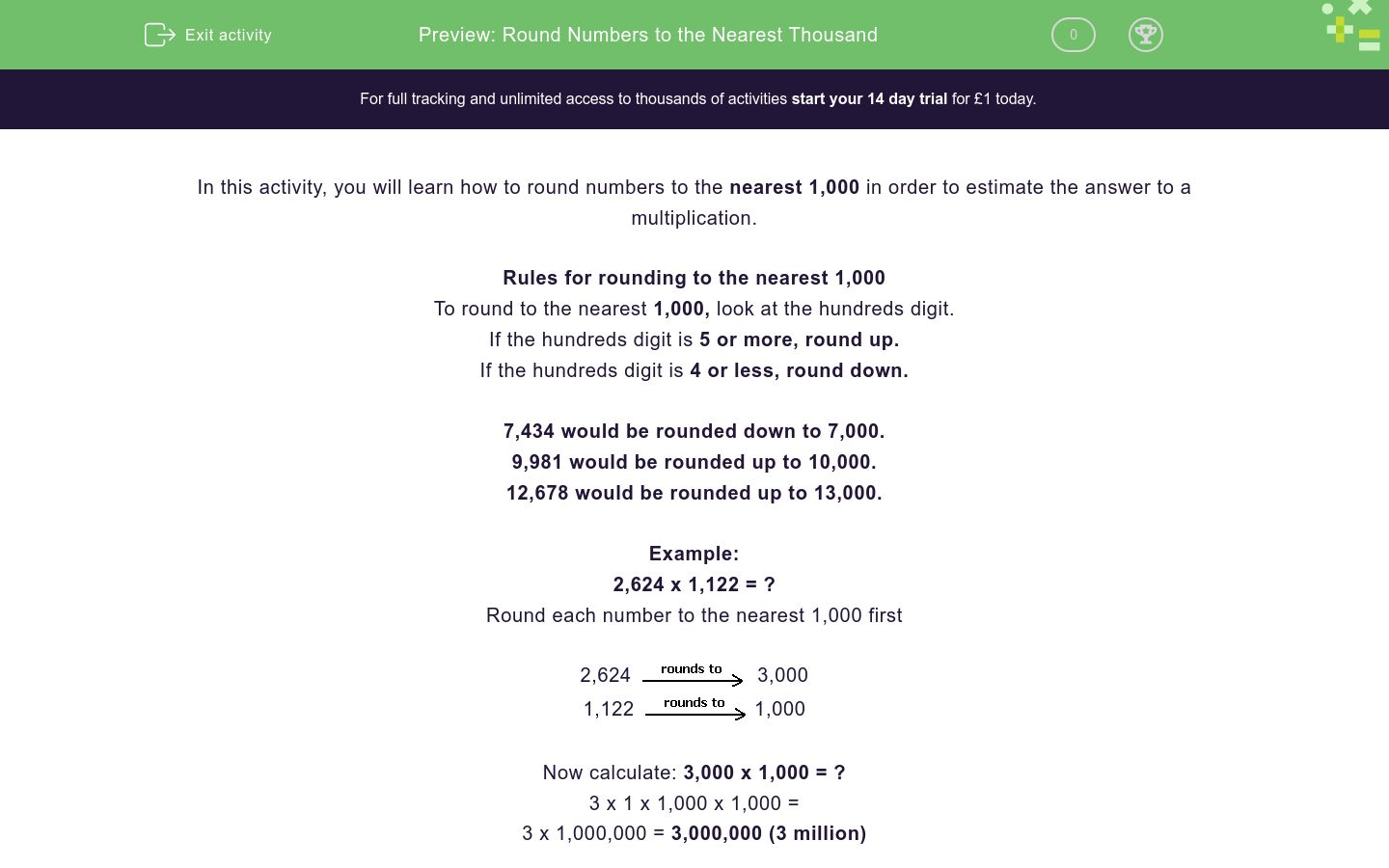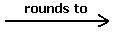# Round Numbers to the Nearest Thousand

In this worksheet, students will learn how to round numbers to the nearest thousand in order to estimate the answer to a calculation.Key stage:  KS 2

Curriculum topic:   Number: Addition and Subtraction

Curriculum subtopic:   Use Rounding for Answers/Accuracy

Difficulty level:### QUESTION 1 of 10

In this activity, you will learn how to round numbers to the nearest 1,000 in order to estimate the answer to a multiplication.

Rules for rounding to the nearest 1,000

To round to the nearest 1,000, look at the hundreds digit.

If the hundreds digit is 5 or more, round up.

If the hundreds digit is 4 or less, round down.

7,434 would be rounded down to 7,000.

9,981 would be rounded up to 10,000.

12,678 would be rounded up to 13,000.

Example:

2,624 x 1,122 = ?

Round each number to the nearest 1,000 first

2,6243,000

1,1221,000

Now calculate: 3,000 x 1,000 = ?

3 x 1 x 1,000 x 1,000 =

3 x 1,000,000 = 3,000,000 (3 million)

Estimate the answer by rounding the numbers to the nearest 1,000 before multiplying to find C.

1,742 x 1,062 =A   x   B  =  C

## Column B

A
2,000
B
1,000
C
2,000,000

Estimate the answer by rounding the numbers to the nearest 1,000 before adding to find C.

8,645 + 1,926 =A   x   B  =  C

## Column B

A
2,000
B
11,000
C
9,000

Estimate the answer by rounding the numbers to the nearest 1,000 before subtracting to find C.

19,864 - 4,111 =A   x   B  =  C

## Column B

A
4,000
B
20,000
C
16,000

Using the same estimation method what is the following equation rounded up to the nearest 1000?

19,648 + 13,762 = ?

32,000

31,000

34,000

30,000

Using the same estimation method, calculate the answer by rounding the numbers to the nearest 1000 before multiplying.

4,264 x 1,942 = ?

18,784 - 16,922 = ?

2,100

2,010

1,990

2,000

John is car shopping, he wants to buy two cars.

He is told that the red car he likes is £32,880 and the green car he likes is £18,323.

John's total budget is £70,000.

Round the cost of each car to the nearest 1,000 and estimate if John has enough money.

Does John have enough money to buy both cars?

Yes

No

Reuben is car shopping and is also trying to buy two cars.

He is told that the purple car he would like is £37,255.

Reuben's total budget is £70,000.

Will he be able to buy two purple cars?

Round the cost of each car to the nearest 1,000 and estimate if Reuben has enough money.

Is Reuben able to buy both cars?

No

Yes

A taxi driver is car shopping and wants to buy a fleet of new cars for his firm.

He likes a blue car that costs £12,154.

He decides to buy seven of the blue cars.

Round the cost of each car to the nearest 1,000 and estimate how much the taxi driver will spend in total.

No

Yes

A bike costs £23,512 and a yellow car costs £62,532

Round the cost of each vehicle to the nearest 100 and estimate the cost of buying either the bikes or the cars.

Will it cost more to buy four bikes or two yellow cars?

Four Bikes

Two Yellow Cars

• Question 1

Estimate the answer by rounding the numbers to the nearest 1,000 before multiplying to find C.

1,742 x 1,062 =A   x   B  =  C

## Column B

A
2,000
B
1,000
C
2,000,000
EDDIE SAYS
When rounded to the nearest 1,000 the calculation is shown as 2,000 x 1,000 = 2,000,000.
• Question 2

Estimate the answer by rounding the numbers to the nearest 1,000 before adding to find C.

8,645 + 1,926 =A   x   B  =  C

## Column B

A
9,000
B
2,000
C
11,000
EDDIE SAYS
When rounded to the nearest 1,000, the calculation is shown as 9,000 + 2,000 = 11,000 You’re making great progress!
• Question 3

Estimate the answer by rounding the numbers to the nearest 1,000 before subtracting to find C.

19,864 - 4,111 =A   x   B  =  C

## Column B

A
20,000
B
4,000
C
16,000
EDDIE SAYS
When rounded to the nearest 1,000 the calculation is shown as 20,000 - 4,000 = 16,000 When rounding 19,864 to the nearest 1,000 we round up as the hundreds digit is more than 5, this means the number is then rounded up to 20,000.
• Question 4

Using the same estimation method what is the following equation rounded up to the nearest 1000?

19,648 + 13,762 = ?

34,000
EDDIE SAYS
The rounded calculation to the nearest 1,000 becomes, 20,000 + 14,000 = 34,000.
• Question 5

Using the same estimation method, calculate the answer by rounding the numbers to the nearest 1000 before multiplying.

4,264 x 1,942 = ?

EDDIE SAYS
How did you find rounding to the nearest 1,000 to solve this multiplication? 4,000 x 2,000 = 4 x 2 x 1,000 x 1,000 = 8 x 1,000,000 = 8,000,000
• Question 6

18,784 - 16,922 = ?

2,000
EDDIE SAYS
19,000 - 17,000 = 2,000 Are you feeling more confident?
• Question 7

John is car shopping, he wants to buy two cars.

He is told that the red car he likes is £32,880 and the green car he likes is £18,323.

John's total budget is £70,000.

Round the cost of each car to the nearest 1,000 and estimate if John has enough money.

Does John have enough money to buy both cars?

Yes
EDDIE SAYS
John does have enough money to buy the two cars. The calculation, after rounding, becomes 33,000 + 18,000 = £51,000 John will still have £19,000 left after buying both cars.
• Question 8

Reuben is car shopping and is also trying to buy two cars.

He is told that the purple car he would like is £37,255.

Reuben's total budget is £70,000.

Will he be able to buy two purple cars?

Round the cost of each car to the nearest 1,000 and estimate if Reuben has enough money.

Is Reuben able to buy both cars?

No
EDDIE SAYS
Reuben does not have enough money to buy the two cars. The rounded calculation is 37,000 + 37,000 = £7, 000 He has £4,000 too little to buy 2 purple cars.
• Question 9

A taxi driver is car shopping and wants to buy a fleet of new cars for his firm.

He likes a blue car that costs £12,154.

He decides to buy seven of the blue cars.

Round the cost of each car to the nearest 1,000 and estimate how much the taxi driver will spend in total.

EDDIE SAYS
£12,154 rounded to the nearest 1000 = £12,000 £12,000 x 7 = £84,000 The taxi driver will spend approximately £84,000. Is this getting easier?
• Question 10

A bike costs £23,512 and a yellow car costs £62,532

Round the cost of each vehicle to the nearest 100 and estimate the cost of buying either the bikes or the cars.

Will it cost more to buy four bikes or two yellow cars?

Two Yellow Cars
EDDIE SAYS
Bike cost = £24,000 x 4 = £96,000 Yellow car cost = £63,000 x 2 = £1,250 Therefore, it costs more to buy two yellow cars than it does, four purple bikes.
---- OR ----

Sign up for a £1 trial so you can track and measure your child's progress on this activity.

### What is EdPlace?

We're your National Curriculum aligned online education content provider helping each child succeed in English, maths and science from year 1 to GCSE. With an EdPlace account you’ll be able to track and measure progress, helping each child achieve their best. We build confidence and attainment by personalising each child’s learning at a level that suits them.

Get started